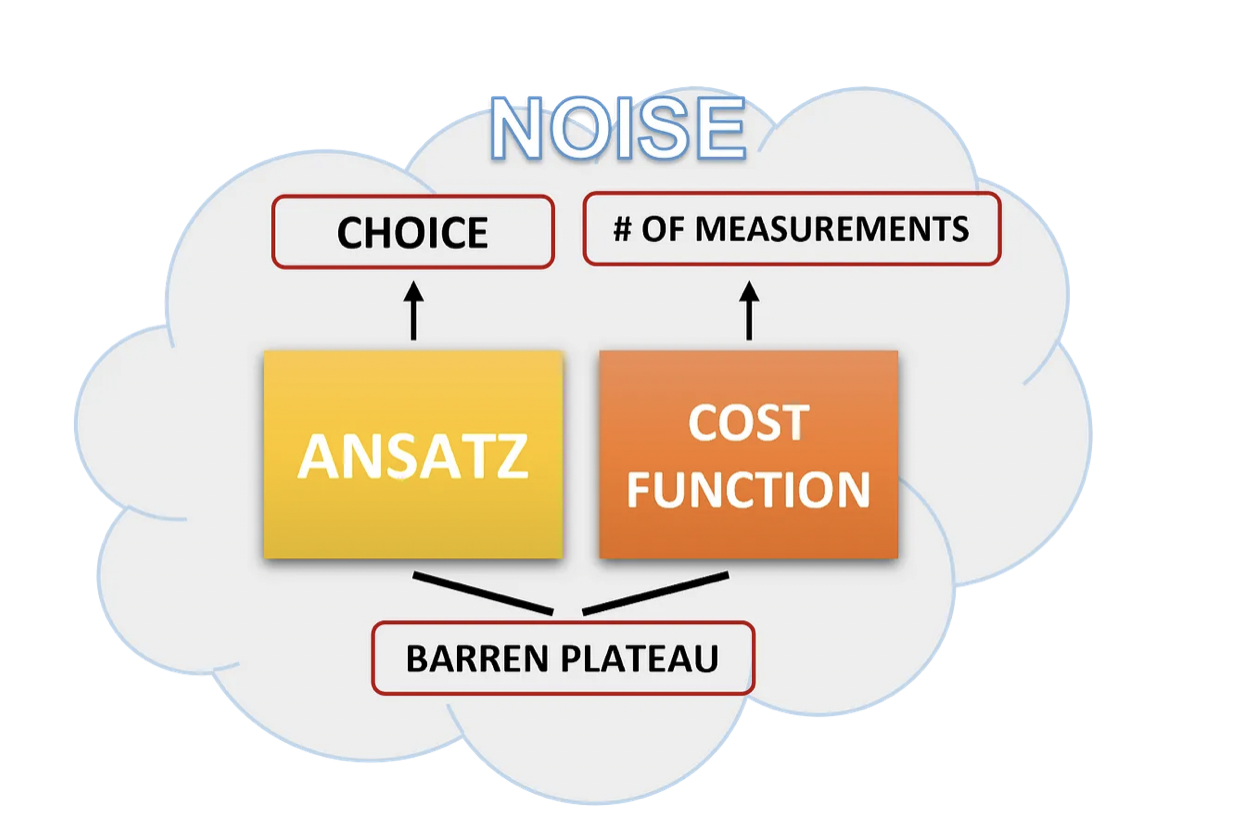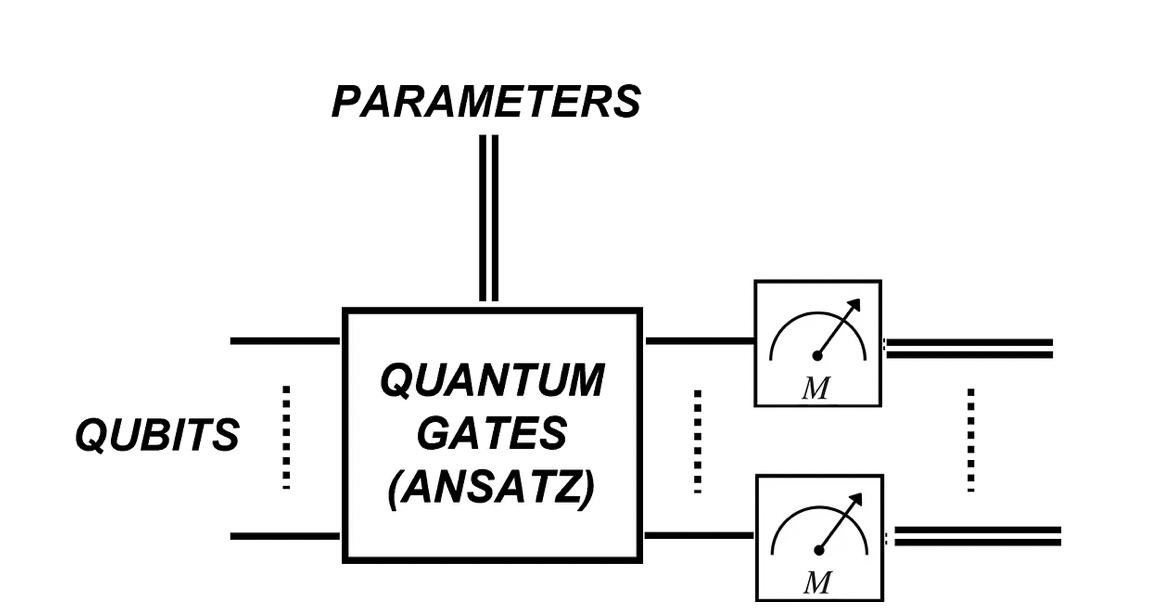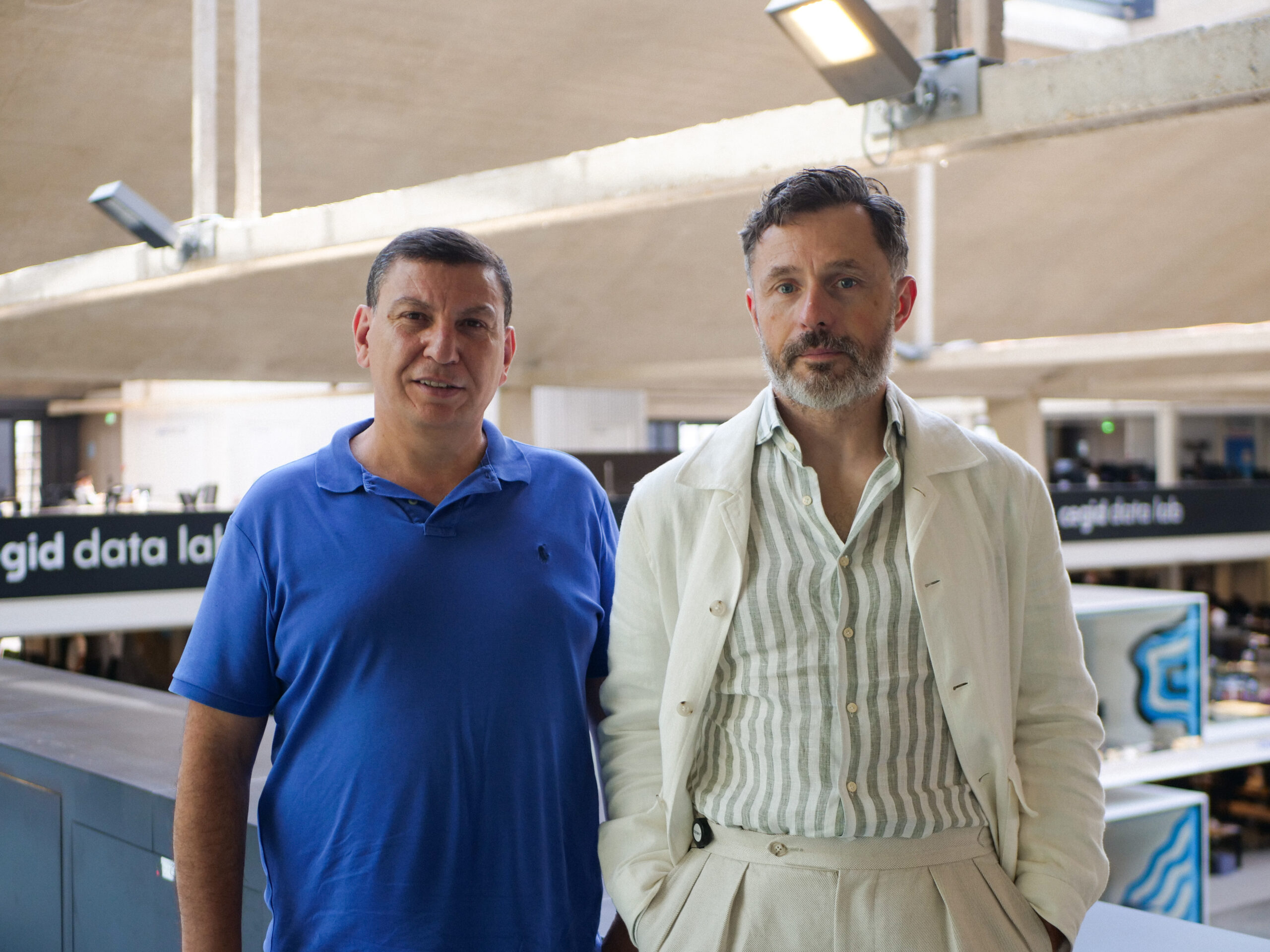# What stands in the way of variational quantum algorithms toward the quantum advantage

By
March 30, 2022
11 minutesWe are living in the exciting era of the first available quantum computers, complex devices which aim at tackling problems by playing by the rules of quantum mechanics. Although still imperfect (too small, too noisy, too error-prone…) they are the result of years and years of research and of the great effort of scientists all over the world, so instead of focusing on what they cannot do, let’s see what they CAN do!

Those imperfect computers are more precisely called Noisy Intermediate-Scale Quantum devices (NISQ) . To make use of any kind of computer, we need algorithms to run on them, so in this case, we need algorithms suitable for NISQ machines. The most known and used ones are the so-called Variational Quantum Algorithms (VQA) , whose features and main challenges will be outlined in the following. The first question that comes to mind is then: where do they originate from?

## The variational principle

The variational method in quantum mechanics is an approximation method based on a theorem, the so-called variational principle, which is very useful to find approximations to the ground state energy of a system, that is the expectation value of the corresponding Hamiltonian in the ground state. The statement of the theorem can be expressed with a simple formula:Variational theorem: the ground state energy is always less or equal than any expectation value of the Hamiltonian in any trial parametrized state.

or in words, as Any (parametrized) trial wavefunction will always give an expectation value larger than or equal to the ground state energy, since, by definition, the ground state wavefunction has the lowest energy!

Variational quantum algorithms make use of this principle: it can be exploited exactly as written above when the interest is finding the ground state eigenvalue and eigenvector of a Hamiltonian; knowing the structure of a molecule can indeed be very important in different areas of science, as in quantum chemistry, e.g. to improve drug discovery, or in material science, e.g. to analyze superconductivity properties. In a more general way, one can instead translate different problems into Hamiltonian ones or also define alternative functions, called cost functions, to parametrize and minimize.

But why are these algorithms suited for NISQ devices?

## Hybrid Quantum/Classical Algorithms

If we were to apply the variational method to a system of N qubits with a generical trial wavefunction written classically, we would need an exponential number, in N, of complex numbers. What if we delegate this hard task to our first-generation quantum computers? Well, it turns out that this is a very good idea! A quantum computer can write a generic state with less than an exponential number of parameters, through a parametrized quantum circuit (PQC), called the Ansatz of the VQA. But what is even better, is to make NISQ devices cooperate with our (very powerful) classical computers: the result is an example of what we will call a hybrid algorithm. The word hybrid in this case refers to assigning different parts of our algorithm to the quantum or classical device, based on the different strengths of the two machines. Once the state is prepared, the expectation value of the observable chosen as the cost function can be calculated, performing many measurements to obtain a good estimate. The quantum measurements performed in NISQ devices are usually restricted to the computational basis. At this point, the classical computer comes into play with its powerful ways of optimizing the variational parameters; several different methods are known, both gradient-based and gradient-free. The optimized parameters are finally given back to the quantum computer to re-run the PQC, thus starting again the hybrid loop.

It can be useful to emphasize how the quantum and classical devices communicate, or in other words how classical and quantum information are exchanged in such hybrid algorithms. The classical parameters are converted into quantum information since they parametrize quantum gates, which in turn produce a quantum state. Viceversa, the quantum information contained in the state is translated back into classical as a result of quantum measurement, as depicted in the figure below.Quantum and classical information in a circuit representation (the single line represents a qubit, while the double line is a classical bit).

Among the several examples of applications of VQAs, we can find the Variational Quantum Eigensolver (VQE) in the context of quantum chemistry [3,4,5,6], the Quantum Approximate Optimization Algorithm (QAOA) in the context of optimization, and combinatorial problems [7,8,9,10] and the Variational Quantum Linear Solver (VQLS) in the context of linear algebra [11,12,13,14]. All these algorithms have attracted a lot of attention and quite a big amount of works have advanced in these directions, showing e.g., in quantum chemistry problems, the reduction of coherence times requirements for the calculation of ground-state molecular energy , the experimental implementation of VQE with up to six qubits and more than one hundred Pauli terms  or the extension of VQE to the calculation of transition energies between the ground state and low excited states of a molecule .

Nevertheless, we still have not experienced a drastic change in the solution of complex problems and we keep facing not only hardware limitations but also software ones (see e.g. ). Why is that?

Let’s get an idea!

One of the reasons why VQAs are attractive is their being task-oriented algorithms. Their modular structure allows one to change each piece (ansatz, cost function, optimizer) depending on the problem at hand. The wide exploration of several different applications has shed light on some common challenges that can arise in running such algorithms, affecting their efficiency and their solution accuracy . We will discuss some of them, neglecting the classical part and only focusing on the quantum one (for an example of different performances of classical optimizers see e.g. ). As depicted in the figure below, noise affects the whole algorithm implementation, while some of the problems can arise only for a specific part of it.

Expressibility and entangling power The choice of the Ansatz in a VQA is a crucial one, and the performance of the algorithm can strongly depend on it, so it would be nice to have some general figures of merit in order to evaluate how good the chosen Ansatz is, and if it is the right pick for the specific use case considered. Two quantities were recently proposed in . The first one, called expressibility, represents the capability of a quantum circuit of generating states in the Hilbert space; the ideal case would be a uniform distribution in the state space. Low expressibility means the possibility of exploring only a limited set of states, thus the higher the expressibility, the larger the set of accessible states. The second one is instead the entangling power of a quantum circuit, which refers to how entangled are the states generated by it; this is done by averaging a chosen entanglement measure over a certain number of parametrized samples states. Tests are needed to verify whether these two quantities are indeed good figures of merit to discriminate among different Ansätze; in  it was shown for instance that in the presence of noise, the expressibility of the Ansatz chosen for the VQE studied did not perform well as a criterion to choose an optimal circuit, since it resulted not correlated to the performance of the algorithm.

Barren Plateau Some cost function landscapes could present a flat region, known as the Barren plateau, and they could become flatter as the number of qubits in the circuit increases; the problem, in this case, is that one needs an exponentially large precision to find a minimization direction, potentially compromising the possibility of gaining an advantage from the VQA compared to a fully classical algorithm, to which an exponential scaling is usually associated. It was shown in  that randomly initialized PQC can lead to Barren plateaus, but they can also depend on the choice of the cost function, whether it is global or local (relative to the whole register or to the single qubit), as discussed in .

Number of measurements A very well-known problem in the estimation of the expectation value of the cost function is the limited number of available resources, more specifically the number of quantum measurements that one can perform in order to reach enough precision, which could be too many to be performed in the time available. This again represents a threat to the potential quantum advantage of a VQA. Moreover, what is usually done in current algorithms, e.g. for a Hamiltonian in a VQE, is to decompose the operator in Pauli terms and to evaluate the sum of the single term expectation values; depending on the complexity of the operator, this decomposition could contain many terms, with a corresponding increase in the number of measurements.

Noise It is well-known that noise is the number one enemy for quantum hardwares since it leads any quantum system to decohere (after a sufficiently long time). It is then only natural to ask how it affects quantum algorithms, especially the ones running on NISQ devices, which cannot implement full quantum error correction because of the restricted number of qubits. The consequence of this is limited circuit depth to avoid as much as possible the propagation of gate errors. Noise can also be responsible for some of the VQA problems discussed above: it can for instance induce Barren plateaus  independently of the Ansatz used, affecting the trainability of the cost function, or it can compromise the final value of the cost function, which is an important quantity in some VQAs.

All the above-mentioned problems could sound overwhelming, but if we look at them as challenges, then we are left with a bunch of new open questions, which increase interest and curiosity in trying and finding an answer to them.

Much different research directions have started addressing the problems above, offering possible solutions and new insights, pursuing the final goal of quantum advantage in practical applications. For instance, the choice of the optimal Ansatz should be done keeping always in mind the noise model and the desired use case one wants to solve since the performance of the circuit will depend on both these factors . The choice of the Ansatz also is crucial for avoiding Barren plateaus, and parameters initialization strategies have been proposed to reduce randomization . The number of measurements to estimate an expectation value could instead be reduced by considering commuting (e.g. which can be measured simultaneously) subsets of Pauli strings in the decomposition of the observable ; moreover, this could be improved by optimizing the allocation of shots among the Pauli operators (the ones with smaller coefficients contribute less to the variance) .

Finally, the question remains: are VQAs noise resilient? At present knowledge, the answer is that it depends on the type of noise; if it is coherent, like a unitary operation which instead of performing a rotation of an angle A results in a rotation of A+Err, then the VQA is not affected by it and the parameter optimization will adjust itself . When the noise is incoherent (e.g. decoherence), only a few results are available (see e.g. ) and further investigations are needed to get a clearer picture.

## References

 Preskill, J. (2018). Quantum computing in the NISQ era and beyond. Quantum, 2, 79.

 Cerezo, M., Arrasmith, A., Babbush, R., Benjamin, S. C., Endo, S., Fujii, K., … & Coles, P. J. (2021). Variational quantum algorithms. Nature Reviews Physics, 3(9), 625–644.

 Tilly, J., Chen, H., Cao, S., Picozzi, D., Setia, K., Li, Y., … & Tennyson, J. (2021). The Variational Quantum Eigensolver: a review of methods and best practices. arXiv preprint arXiv:2111.05176.

 Wang, D., Higgott, O., & Brierley, S. (2019). Accelerated variational quantum eigensolver. Physical review letters, 122(14), 140504.

 Kandala, A., Mezzacapo, A., Temme, K., Takita, M., Brink, M., Chow, J. M., & Gambetta, J. M. (2017). Hardware-efficient variational quantum eigensolver for small molecules and quantum magnets. Nature, 549(7671), 242–246.

 Liu, J. G., Zhang, Y. H., Wan, Y., & Wang, L. (2019). Variational quantum eigensolver with fewer qubits. Physical Review Research, 1(2), 023025

 Niu, M. Y., Lu, S., & Chuang, I. L. (2019). Optimizing qaoa: Success probability and runtime dependence on circuit depth. arXiv preprint arXiv:1905.12134.

 Willsch, M., Willsch, D., Jin, F., De Raedt, H., & Michielsen, K. (2020). Benchmarking the quantum approximate optimization algorithm. Quantum Information Processing, 19(7), 1–24.

 Zhou, L., Wang, S. T., Choi, S., Pichler, H., & Lukin, M. D. (2020). Quantum approximate optimization algorithm: Performance, mechanism, and implementation on near-term devices. Physical Review X, 10(2), 021067.

 Shaydulin, R., & Alexeev, Y. (2019, October). Evaluating quantum approximate optimization algorithm: A case study. In 2019 tenth international green and sustainable computing conference (IGSC) (pp. 1–6). IEEE.

 Bravo-Prieto, C., LaRose, R., Cerezo, M., Subasi, Y., Cincio, L., & Coles, P. J. (2019). Variational quantum linear solver. arXiv preprint arXiv:1909.05820.

 Huang, H. Y., Bharti, K., & Rebentrost, P. (2019). Near-term quantum algorithms for linear systems of equations. arXiv preprint arXiv:1909.07344.

 Xu, X., Sun, J., Endo, S., Li, Y., Benjamin, S. C., & Yuan, X. (2021). Variational algorithms for linear algebra. Science Bulletin, 66(21), 2181–2188.

 Patil, H., Wang, Y., & Krstić, P. S. (2022). Variational quantum linear solver with a dynamic ansatz. Physical Review A, 105(1), 012423.

 Peruzzo, A., McClean, J., Shadbolt, P., Yung, M. H., Zhou, X. Q., Love, P. J., … & O’brien, J. L. (2014). A variational eigenvalue solver on a photonic quantum processor. Nature communications, 5(1), 1–7.

 Parrish, R. M., Hohenstein, E. G., McMahon, P. L., & Martínez, T. J. (2019). Quantum computation of electronic transitions using a variational quantum eigensolver. Physical review letters, 122(23), 230401.

 Guerreschi, G. G., & Matsuura, A. Y. (2019). QAOA for Max-Cut requires hundreds of qubits for quantum speed-up. Scientific reports, 9(1), 1–7.

 Pellow-Jarman, A., Sinayskiy, I., Pillay, A., & Petruccione, F. (2021). A comparison of various classical optimizers for a variational quantum linear solver. Quantum Information Processing, 20(6), 1–14.

 Sim, S., Johnson, P. D., & Aspuru‐Guzik, A. (2019). Expressibility and entangling capability of parameterized quantum circuits for hybrid quantum‐classical algorithms. Advanced Quantum Technologies, 2(12), 1900070.

 Saib, W., Wallden, P., & Akhalwaya, I. (2021, October). The Effect of Noise on the Performance of Variational Algorithms for Quantum Chemistry. In 2021 IEEE International Conference on Quantum Computing and Engineering (QCE) (pp. 42–53). IEEE.

 McClean, J. R., Boixo, S., Smelyanskiy, V. N., Babbush, R., & Neven, H. (2018). Barren plateaus in quantum neural network training landscapes. Nature communications, 9(1), 1–6.

 Cerezo, M., Sone, A., Volkoff, T., Cincio, L., & Coles, P. J. (2021). Cost function dependent barren plateaus in shallow parametrized quantum circuits. Nature communications, 12(1), 1–12.

 Wang, S., Fontana, E., Cerezo, M., Sharma, K., Sone, A., Cincio, L., & Coles, P. J. (2021). Noise-induced barren plateaus in variational quantum algorithms. Nature communications, 12(1), 1–11.

 Volkoff, T., & Coles, P. J. (2021). Large gradients via correlation in random parameterized quantum circuits. Quantum Science and Technology, 6(2), 025008.

 McClean, J. R., Romero, J., Babbush, R., & Aspuru-Guzik, A. (2016). The theory of variational hybrid quantum-classical algorithms. New Journal of Physics, 18(2), 023023.

 Rubin, N. C., Babbush, R., & McClean, J. (2018). Application of fermionic marginal constraints to hybrid quantum algorithms. New Journal of Physics, 20(5), 053020.

 O’Malley, P. J., Babbush, R., Kivlichan, I. D., Romero, J., McClean, J. R., Barends, R., … & Martinis, J. M. (2016). Scalable quantum simulation of molecular energies. Physical Review X, 6(3), 031007.

 Sharma, K., Khatri, S., Cerezo, M., & Coles, P. J. (2020). Noise resilience of variational quantum compiling. New Journal of Physics, 22(4), 043006.